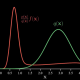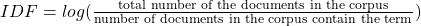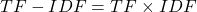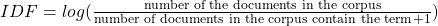###### Steps to package and publish Python codes to PyPI (pip)
2022-07-28###### A simple tutorial on Sampling Importance and Monte Carlo with Python codes
2022-08-01

Term Frequency – Inverse Document Frequency (TF-IDF) is a popular statistical technique utilized in natural language processing and information retrieval to assess a term’s significance in a document in comparison to a group of documents, known as a corpus. The technique employs a text vectorization process to transform words in a text document into numerical values that denote their importance. Various scoring methods exist for text vectorization, with TF-IDF being among the most prevalent. As its name implies, TF-IDF vectorizes/scores a word by multiplying the word’s Term Frequency (TF) with the Inverse Document Frequency (IDF). You can find the codes of this post on my Github.

Term Frequency: TF of a term or word is the number of times the term appears in a document compared to the total number of words in the document.Inverse Document Frequency: IDF of a term reflects the proportion of documents in the corpus that contain the term. Words unique to a small percentage of documents (e.g., technical jargon terms) receive higher importance values than words common across all documents (e.g., a, the, and).The TF-IDF of a term is calculated by multiplying TF and IDF scores.Translated into plain English, the importance of a term is high when it occurs a lot in a given document and rarely in others. In short, commonality within a document measured by TF is balanced by rarity between documents measured by IDF. The resulting TF-IDF score reflects the importance of a term for a document in the corpus.

TF-IDF is useful in many natural language processing applications. For example, Search Engines use TF-IDF to rank the relevance of a document for a query. TF-IDF is also employed in text classification, text summarization, and topic modeling.

Note that there are some different approaches to calculating the IDF score. The base 10 logarithm is often used in the calculation. However, some libraries use a natural logarithm. In addition, one can be added to the denominator as follows in order to avoid division by zero.## Numerical Example

Imagine the term t appears 20 times in a document that contains a total of 100 words. The Term Frequency (TF) of t can be calculated as follow:

TF=20/100=0.2

Assume a collection of related documents containing 10,000 documents. If 100 documents out of 10,000 documents contain the term t, the Inverse Document Frequency (IDF) of t can be calculated as follows

IDF=log(10000/100)=2

Using these two quantities, we can calculate the TF-IDF score of the term t for the document.

TF-IDF=0.2×2=0.4

## Python Implementation

Some popular python libraries have a function to calculate TF-IDF. The popular machine learning library Sklearn has TfidfVectorizer() function (docs).

We will write a TF-IDF function from scratch using the standard formula given above, but we will not apply any preprocessing operations such as stop words removal, stemming, punctuation removal, or lowercasing. It should be noted that the result may be different when using a native function built into a library.

import pandas as pd
import numpy as np


First, let’s construct a small corpus.

corpus = ['data science is one of the most important fields of science',
'this is one of the best data science courses',
'data scientists analyze data' ]


Next, we’ll create a word set for the corpus:

words_set = set()

for doc in  corpus:
words = doc.split(' ')
words_set = words_set.union(set(words))

print('Number of words in the corpus:',len(words_set))
print('The words in the corpus: \n', words_set)


OUT:

Number of words in the corpus: 14
The words in the corpus:
{'important', 'scientists', 'best', 'courses', 'this', 'analyze', 'of', 'most', 'the', 'is', 'science', 'fields', 'one', 'data'}


### Computing Term Frequency

Now we can create a dataframe by the number of documents in the corpus and the word set, and use that information to compute the term frequency (TF):

n_docs = len(corpus)         #·Number of documents in the corpus
n_words_set = len(words_set) #·Number of unique words in the

df_tf = pd.DataFrame(np.zeros((n_docs, n_words_set)), columns=words_set)

# Compute Term Frequency (TF)
for i in range(n_docs):
words = corpus[i].split(' ') # Words in the document
for w in words:
df_tf[w][i] = df_tf[w][i] + (1 / len(words))

df_tf


OUT:

The dataframe above shows we have a column for each word and a row for each document. This shows the frequency of each word in each document.

### Computing Inverse Document Frequency

Now, we’ll compute the inverse document frequency (IDF):

print("IDF of: ")

idf = {}

for w in words_set:
k = 0    # number of documents in the corpus that contain this word

for i in range(n_docs):
if w in corpus[i].split():
k += 1

idf[w] =  np.log10(n_docs / k)

print(f'{w:>15}: {idf[w]:>10}' )


OUT:

IDF of:
important: 0.47712125471966244
scientists: 0.47712125471966244
best: 0.47712125471966244
courses: 0.47712125471966244
this: 0.47712125471966244
analyze: 0.47712125471966244
of: 0.17609125905568124
most: 0.47712125471966244
the: 0.17609125905568124
is: 0.17609125905568124
science: 0.17609125905568124
fields: 0.47712125471966244
one: 0.17609125905568124
data:        0.0


### Putting it Together: Computing TF-IDF

Since we have TF and IDF now, we can compute TF-IDF:

df_tf_idf = df_tf.copy()

for w in words_set:
for i in range(n_docs):
df_tf_idf[w][i] = df_tf[w][i] * idf[w]

df_tf_idf


OUT:

Notice that “data” has an IDF of 0 because it appears in every document. As a result, “is” is not considered to be an important term in this corpus. This will change slightly in the following sklearn implementation, where “data” will be non-zero.

## TF-IDF Using scikit-learn

First, we need to import sklearn TfidfVectorizer:

from sklearn.feature_extraction.text import TfidfVectorizer


We need to instantiate the class first, then we can call the fit_transform method on our test corpus. This will perform all of the calculations we performed above.

tr_idf_model  = TfidfVectorizer()
tf_idf_vector = tr_idf_model.fit_transform(corpus)


After vectorizing the corpus by the function, a sparse matrix is obtained.

Here’s the current shape of the matrix:

print(type(tf_idf_vector), tf_idf_vector.shape)
# <class'scipy.sparse.csr.csr_matrix'>(3,14)


And we can convert to a regular array to get a better idea of the values:

tf_idf_array = tf_idf_vector.toarray()

print(tf_idf_array)


OUT:

[[0.         0.         0.         0.18952581 0.32089509 0.32089509
0.24404899 0.32089509 0.48809797 0.24404899 0.48809797 0.
0.24404899 0.        ]
[0.         0.40029393 0.40029393 0.23642005 0.         0.
0.30443385 0.         0.30443385 0.30443385 0.30443385 0.
0.30443385 0.40029393]
[0.54270061 0.         0.         0.64105545 0.         0.
0.         0.         0.         0.         0.         0.54270061
0.         0.        ]]


It’s now very straightforward to obtain the original terms in the corpus by using get_feature_names_out:

words_set = tr_idf_model.get_feature_names_out()

print(words_set)


OUT:

['analyze', 'best', 'courses', 'data', 'fields', 'important', 'is', 'most', 'of', 'one', 'science', 'scientists', 'the', 'this']


Finally, we’ll create a dataframe to better show the TF-IDF scores of each document:

df_tf_idf = pd.DataFrame(tf_idf_array, columns = words_set)

df_tf_idf


OUT:

As you can see from the output above, the TF-IDF scores are different than the scores obtained by the manual process we used earlier. This difference is due to the sklearn implementation of TF-IDF, which uses a slightly different formula. For more details, you can learn more about how sklearn calculates TF-IDF term weighting here.